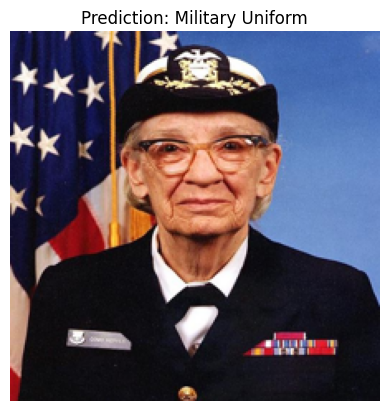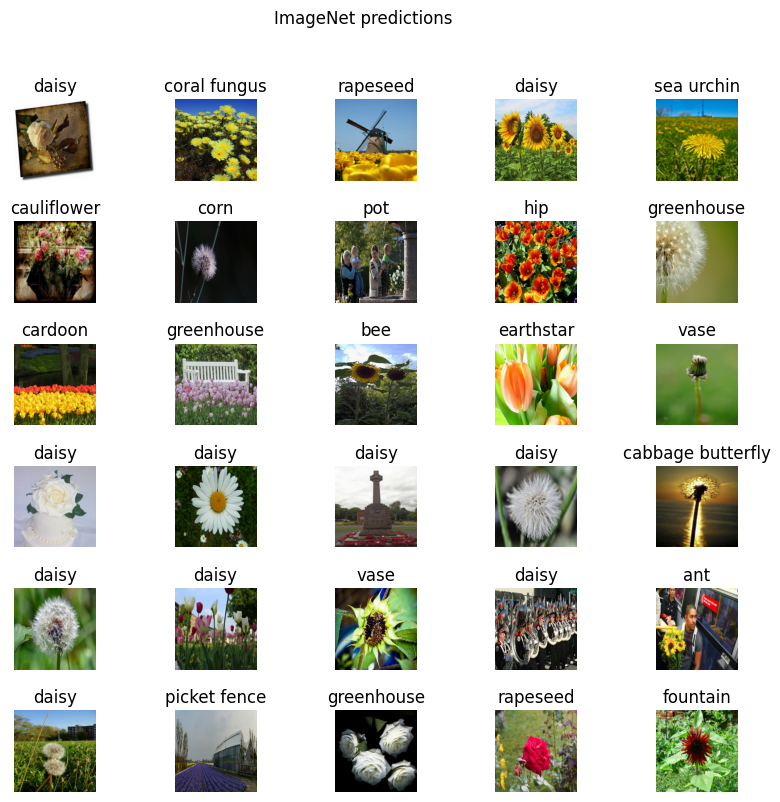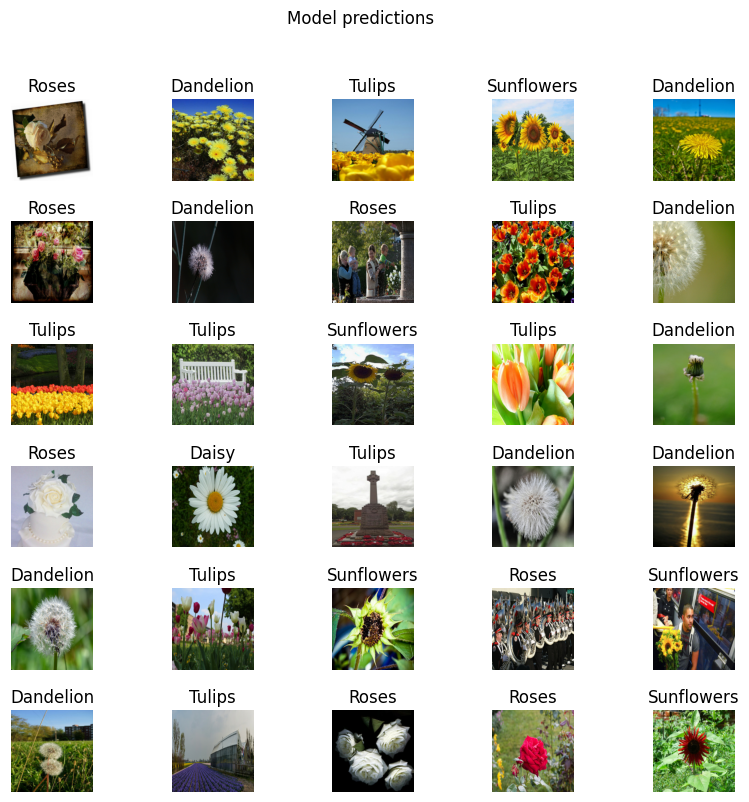# Transfer learning with TensorFlow Hub

TensorFlow Hub is a repository of pre-trained TensorFlow models.

This tutorial demonstrates how to:

1. Use models from TensorFlow Hub with `tf.keras`.
2. Use an image classification model from TensorFlow Hub.
3. Do simple transfer learning to fine-tune a model for your own image classes.

## Setup

``````import numpy as np
import time

import PIL.Image as Image
import matplotlib.pylab as plt

import tensorflow as tf
import tensorflow_hub as hub

import datetime

``````
```2023-09-01 02:44:48.629530: E tensorflow/compiler/xla/stream_executor/cuda/cuda_dnn.cc:9342] Unable to register cuDNN factory: Attempting to register factory for plugin cuDNN when one has already been registered
2023-09-01 02:44:48.629576: E tensorflow/compiler/xla/stream_executor/cuda/cuda_fft.cc:609] Unable to register cuFFT factory: Attempting to register factory for plugin cuFFT when one has already been registered
2023-09-01 02:44:48.629608: E tensorflow/compiler/xla/stream_executor/cuda/cuda_blas.cc:1518] Unable to register cuBLAS factory: Attempting to register factory for plugin cuBLAS when one has already been registered
WARNING:tensorflow:From /tmpfs/src/tf_docs_env/lib/python3.9/site-packages/tensorflow/python/ops/distributions/distribution.py:259: ReparameterizationType.__init__ (from tensorflow.python.ops.distributions.distribution) is deprecated and will be removed after 2019-01-01.
Instructions for updating:
The TensorFlow Distributions library has moved to TensorFlow Probability (https://github.com/tensorflow/probability). You should update all references to use `tfp.distributions` instead of `tf.distributions`.
WARNING:tensorflow:From /tmpfs/src/tf_docs_env/lib/python3.9/site-packages/tensorflow/python/ops/distributions/bernoulli.py:165: RegisterKL.__init__ (from tensorflow.python.ops.distributions.kullback_leibler) is deprecated and will be removed after 2019-01-01.
Instructions for updating:
The TensorFlow Distributions library has moved to TensorFlow Probability (https://github.com/tensorflow/probability). You should update all references to use `tfp.distributions` instead of `tf.distributions`.
```

## An ImageNet classifier

You'll start by using a classifier model pre-trained on the ImageNet benchmark dataset—no initial training required!

Select a MobileNetV2 pre-trained model from TensorFlow Hub and wrap it as a Keras layer with `hub.KerasLayer`. Any compatible image classifier model from TensorFlow Hub will work here, including the examples provided in the drop-down below.

``````mobilenet_v2 ="https://tfhub.dev/google/tf2-preview/mobilenet_v2/classification/4"

classifier_model = mobilenet_v2
``````
``````IMAGE_SHAPE = (224, 224)

classifier = tf.keras.Sequential([
hub.KerasLayer(classifier_model, input_shape=IMAGE_SHAPE+(3,))
])
``````

### Run it on a single image

``````grace_hopper = tf.keras.utils.get_file('image.jpg','https://storage.googleapis.com/download.tensorflow.org/example_images/grace_hopper.jpg')
grace_hopper = Image.open(grace_hopper).resize(IMAGE_SHAPE)
grace_hopper
``````
```Downloading data from https://storage.googleapis.com/download.tensorflow.org/example_images/grace_hopper.jpg
61306/61306 [==============================] - 0s 0us/step
`````````grace_hopper = np.array(grace_hopper)/255.0
grace_hopper.shape
``````
```(224, 224, 3)
```

Add a batch dimension (with `np.newaxis`) and pass the image to the model:

``````result = classifier.predict(grace_hopper[np.newaxis, ...])
result.shape
``````
```1/1 [==============================] - 1s 1s/step
(1, 1001)
```

The result is a 1001-element vector of logits, rating the probability of each class for the image.

The top class ID can be found with `tf.math.argmax`:

``````predicted_class = tf.math.argmax(result, axis=-1)
predicted_class
``````
```<tf.Tensor: shape=(), dtype=int64, numpy=653>
```

### Decode the predictions

Take the `predicted_class` ID (such as `653`) and fetch the ImageNet dataset labels to decode the predictions:

``````labels_path = tf.keras.utils.get_file('ImageNetLabels.txt','https://storage.googleapis.com/download.tensorflow.org/data/ImageNetLabels.txt')
``````
```Downloading data from https://storage.googleapis.com/download.tensorflow.org/data/ImageNetLabels.txt
10484/10484 [==============================] - 0s 0us/step
```
``````plt.imshow(grace_hopper)
plt.axis('off')
predicted_class_name = imagenet_labels[predicted_class]
_ = plt.title("Prediction: " + predicted_class_name.title())
``````## Simple transfer learning

But what if you want to create a custom classifier using your own dataset that has classes that aren't included in the original ImageNet dataset (that the pre-trained model was trained on)?

To do that, you can:

1. Select a pre-trained model from TensorFlow Hub; and
2. Retrain the top (last) layer to recognize the classes from your custom dataset.

### Dataset

In this example, you will use the TensorFlow flowers dataset:

``````import pathlib

data_file = tf.keras.utils.get_file(
'flower_photos.tgz',
cache_dir='.',
extract=True)

data_root = pathlib.Path(data_file).with_suffix('')
``````
```Downloading data from https://storage.googleapis.com/download.tensorflow.org/example_images/flower_photos.tgz
228813984/228813984 [==============================] - 2s 0us/step
```

First, load this data into the model using the image data off disk with `tf.keras.utils.image_dataset_from_directory`, which will generate a `tf.data.Dataset`:

``````batch_size = 32
img_height = 224
img_width = 224

train_ds = tf.keras.utils.image_dataset_from_directory(
str(data_root),
validation_split=0.2,
subset="training",
seed=123,
image_size=(img_height, img_width),
batch_size=batch_size
)

val_ds = tf.keras.utils.image_dataset_from_directory(
str(data_root),
validation_split=0.2,
subset="validation",
seed=123,
image_size=(img_height, img_width),
batch_size=batch_size
)
``````
```Found 3670 files belonging to 5 classes.
Using 2936 files for training.
Found 3670 files belonging to 5 classes.
Using 734 files for validation.
```

The flowers dataset has five classes:

``````class_names = np.array(train_ds.class_names)
print(class_names)
``````
```['daisy' 'dandelion' 'roses' 'sunflowers' 'tulips']
```

Second, because TensorFlow Hub's convention for image models is to expect float inputs in the `[0, 1]` range, use the `tf.keras.layers.Rescaling` preprocessing layer to achieve this.

``````normalization_layer = tf.keras.layers.Rescaling(1./255)
train_ds = train_ds.map(lambda x, y: (normalization_layer(x), y)) # Where x—images, y—labels.
val_ds = val_ds.map(lambda x, y: (normalization_layer(x), y)) # Where x—images, y—labels.
``````

Third, finish the input pipeline by using buffered prefetching with `Dataset.prefetch`, so you can yield the data from disk without I/O blocking issues.

These are some of the most important `tf.data` methods you should use when loading data. Interested readers can learn more about them, as well as how to cache data to disk and other techniques, in the Better performance with the tf.data API guide.

``````AUTOTUNE = tf.data.AUTOTUNE
train_ds = train_ds.cache().prefetch(buffer_size=AUTOTUNE)
val_ds = val_ds.cache().prefetch(buffer_size=AUTOTUNE)
``````
``````for image_batch, labels_batch in train_ds:
print(image_batch.shape)
print(labels_batch.shape)
break
``````
```(32, 224, 224, 3)
(32,)
2023-09-01 02:45:01.881978: W tensorflow/core/kernels/data/cache_dataset_ops.cc:854] The calling iterator did not fully read the dataset being cached. In order to avoid unexpected truncation of the dataset, the partially cached contents of the dataset  will be discarded. This can happen if you have an input pipeline similar to `dataset.cache().take(k).repeat()`. You should use `dataset.take(k).cache().repeat()` instead.
```

### Run the classifier on a batch of images

Now, run the classifier on an image batch:

``````result_batch = classifier.predict(train_ds)
``````
```92/92 [==============================] - 4s 34ms/step
```
``````predicted_class_names = imagenet_labels[tf.math.argmax(result_batch, axis=-1)]
predicted_class_names
``````
```array(['daisy', 'coral fungus', 'rapeseed', ..., 'daisy', 'daisy',
'birdhouse'], dtype='<U30')
```

Check how these predictions line up with the images:

``````plt.figure(figsize=(10,9))
for n in range(30):
plt.subplot(6,5,n+1)
plt.imshow(image_batch[n])
plt.title(predicted_class_names[n])
plt.axis('off')
_ = plt.suptitle("ImageNet predictions")
``````The results are far from perfect, but reasonable considering that these are not the classes the model was trained for (except for "daisy").

TensorFlow Hub also distributes models without the top classification layer. These can be used to easily perform transfer learning.

Select a MobileNetV2 pre-trained model from TensorFlow Hub. Any compatible image feature vector model from TensorFlow Hub will work here, including the examples from the drop-down menu.

``````mobilenet_v2 = "https://tfhub.dev/google/tf2-preview/mobilenet_v2/feature_vector/4"

feature_extractor_model = mobilenet_v2
``````

Create the feature extractor by wrapping the pre-trained model as a Keras layer with `hub.KerasLayer`. Use the `trainable=False` argument to freeze the variables, so that the training only modifies the new classifier layer:

``````feature_extractor_layer = hub.KerasLayer(
feature_extractor_model,
input_shape=(224, 224, 3),
trainable=False)
``````

The feature extractor returns a 1280-long vector for each image (the image batch size remains at 32 in this example):

``````feature_batch = feature_extractor_layer(image_batch)
print(feature_batch.shape)
``````
```(32, 1280)
```

To complete the model, wrap the feature extractor layer in a `tf.keras.Sequential` model and add a fully-connected layer for classification:

``````num_classes = len(class_names)

model = tf.keras.Sequential([
feature_extractor_layer,
tf.keras.layers.Dense(num_classes)
])

model.summary()
``````
```Model: "sequential_1"
_________________________________________________________________
Layer (type)                Output Shape              Param #
=================================================================
keras_layer_1 (KerasLayer)  (None, 1280)              2257984

dense (Dense)               (None, 5)                 6405

=================================================================
Total params: 2264389 (8.64 MB)
Trainable params: 6405 (25.02 KB)
Non-trainable params: 2257984 (8.61 MB)
_________________________________________________________________
```
``````predictions = model(image_batch)
``````
``````predictions.shape
``````
```TensorShape([32, 5])
```

### Train the model

Use `Model.compile` to configure the training process and add a `tf.keras.callbacks.TensorBoard` callback to create and store logs:

``````model.compile(
loss=tf.keras.losses.SparseCategoricalCrossentropy(from_logits=True),
metrics=['acc'])

log_dir = "logs/fit/" + datetime.datetime.now().strftime("%Y%m%d-%H%M%S")
tensorboard_callback = tf.keras.callbacks.TensorBoard(
log_dir=log_dir,
histogram_freq=1) # Enable histogram computation for every epoch.
``````

Now use the `Model.fit` method to train the model.

To keep this example short, you'll be training for just 10 epochs. To visualize the training progress in TensorBoard later, create and store logs an a TensorBoard callback.

``````NUM_EPOCHS = 10

history = model.fit(train_ds,
validation_data=val_ds,
epochs=NUM_EPOCHS,
callbacks=tensorboard_callback)
``````
```Epoch 1/10
92/92 [==============================] - 10s 76ms/step - loss: 0.7201 - acc: 0.7394 - val_loss: 0.4460 - val_acc: 0.8569
Epoch 2/10
92/92 [==============================] - 6s 62ms/step - loss: 0.3666 - acc: 0.8753 - val_loss: 0.3607 - val_acc: 0.8801
Epoch 3/10
92/92 [==============================] - 6s 62ms/step - loss: 0.2884 - acc: 0.9108 - val_loss: 0.3294 - val_acc: 0.8951
Epoch 4/10
92/92 [==============================] - 6s 62ms/step - loss: 0.2408 - acc: 0.9302 - val_loss: 0.3147 - val_acc: 0.8992
Epoch 5/10
92/92 [==============================] - 6s 62ms/step - loss: 0.2067 - acc: 0.9424 - val_loss: 0.3069 - val_acc: 0.8978
Epoch 6/10
92/92 [==============================] - 6s 63ms/step - loss: 0.1804 - acc: 0.9496 - val_loss: 0.3024 - val_acc: 0.9005
Epoch 7/10
92/92 [==============================] - 6s 62ms/step - loss: 0.1592 - acc: 0.9574 - val_loss: 0.2995 - val_acc: 0.9005
Epoch 8/10
92/92 [==============================] - 6s 63ms/step - loss: 0.1416 - acc: 0.9639 - val_loss: 0.2976 - val_acc: 0.9019
Epoch 9/10
92/92 [==============================] - 6s 63ms/step - loss: 0.1269 - acc: 0.9717 - val_loss: 0.2960 - val_acc: 0.9019
Epoch 10/10
92/92 [==============================] - 6s 62ms/step - loss: 0.1142 - acc: 0.9765 - val_loss: 0.2948 - val_acc: 0.9019
```

Start the TensorBoard to view how the metrics change with each epoch and to track other scalar values:

``````%tensorboard --logdir logs/fit
``````### Check the predictions

Obtain the ordered list of class names from the model predictions:

``````predicted_batch = model.predict(image_batch)
predicted_id = tf.math.argmax(predicted_batch, axis=-1)
predicted_label_batch = class_names[predicted_id]
print(predicted_label_batch)
``````
```1/1 [==============================] - 0s 438ms/step
['roses' 'dandelion' 'tulips' 'sunflowers' 'dandelion' 'roses' 'dandelion'
'roses' 'tulips' 'dandelion' 'tulips' 'tulips' 'sunflowers' 'tulips'
'dandelion' 'roses' 'daisy' 'tulips' 'dandelion' 'dandelion' 'dandelion'
'tulips' 'sunflowers' 'roses' 'sunflowers' 'dandelion' 'tulips' 'roses'
'roses' 'sunflowers' 'tulips' 'sunflowers']
```

Plot the model predictions:

``````plt.figure(figsize=(10,9))

for n in range(30):
plt.subplot(6,5,n+1)
plt.imshow(image_batch[n])
plt.title(predicted_label_batch[n].title())
plt.axis('off')
_ = plt.suptitle("Model predictions")
``````Now that you've trained the model, export it as a SavedModel for reusing it later.

``````t = time.time()

export_path = "/tmp/saved_models/{}".format(int(t))
model.save(export_path)

export_path
``````
```INFO:tensorflow:Assets written to: /tmp/saved_models/1693536373/assets
INFO:tensorflow:Assets written to: /tmp/saved_models/1693536373/assets
'/tmp/saved_models/1693536373'
```

Confirm that you can reload the SavedModel and that the model is able to output the same results:

``````reloaded = tf.keras.models.load_model(export_path)
``````
``````result_batch = model.predict(image_batch)
``````
```1/1 [==============================] - 0s 60ms/step
1/1 [==============================] - 0s 475ms/step
```
``````abs(reloaded_result_batch - result_batch).max()
``````
```0.0
```
``````reloaded_predicted_id = tf.math.argmax(reloaded_result_batch, axis=-1)
``````
```['roses' 'dandelion' 'tulips' 'sunflowers' 'dandelion' 'roses' 'dandelion'
'roses' 'tulips' 'dandelion' 'tulips' 'tulips' 'sunflowers' 'tulips'
'dandelion' 'roses' 'daisy' 'tulips' 'dandelion' 'dandelion' 'dandelion'
'tulips' 'sunflowers' 'roses' 'sunflowers' 'dandelion' 'tulips' 'roses'
'roses' 'sunflowers' 'tulips' 'sunflowers']
```
``````plt.figure(figsize=(10,9))
for n in range(30):
plt.subplot(6,5,n+1)
plt.imshow(image_batch[n])
plt.axis('off')
_ = plt.suptitle("Model predictions")
``````## Next steps

You can use the SavedModel to load for inference or convert it to a TensorFlow Lite model (for on-device machine learning) or a TensorFlow.js model (for machine learning in JavaScript).

Discover more tutorials to learn how to use pre-trained models from TensorFlow Hub on image, text, audio, and video tasks.

[]
[]